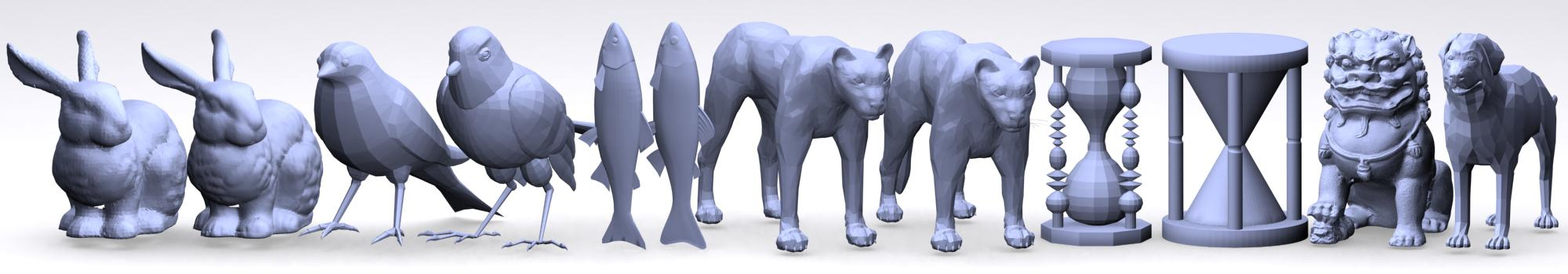Interactive Hausdorff Distance Computation for General Polygonal Models

 Min Tang     Minkyoung Lee     Young J. Kim Department of Computer Science & Engineering Ewha Womans University, Korea

# ACM SIGGRAPH 2009 [Paper] [BibTex] [TechRep][Source Code (Available soon)]

 [Abstract]  We present a simple algorithm to compute the Hausdorff distance between complicated, polygonal models at interactive rates. The algorithm requires no assumptions about the underlying topology and geometry. To avoid the high computational and implementation complexity of exact Hausdorff distance calculation, we approximate the Hausdorff distance within a user-specified error bound. The main ingredient of our approximation algorithm is a novel polygon subdivision scheme, called Voronoi subdivision, combined with culling between the models based on bounding volume hierarchy (BVH). This cross-culling method relies on tight yet simple computation of bounds on the Hausdorff distance, and it discards unnecessary polygon pairs from each of the input models alternatively based on the distance bounds. This algorithm can approximate the Hausdorff distance between polygonal models consisting of tens of thousands triangles with a small error bound in real-time, and outperforms the existing algorithm by more than an order of magnitude. We apply our Hausdorff distance algorithm to the measurement of shape similarity, and the computation of penetration depth for physically-based animation. In particular, the penetration depth computation using Hausdorff distance runs at highly interactive rates for complicated dynamics scene.

Video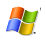(Download 47M)

# Benchmarking Scenarios

 (1) Two-sided Hausdorff Distance Computation    In each benchmarking, two models are placed in space, initially separated by approximately three times their longest dimension. As we translated one of the models toward the other, we computed the two-sided Hausdorff distance between them, measured the timings and averaged them.   In all the benchmarking scenarios, we set the user-specified error bound as 10-4.   In all the figures, the green line denotes the Hausdorff distance result.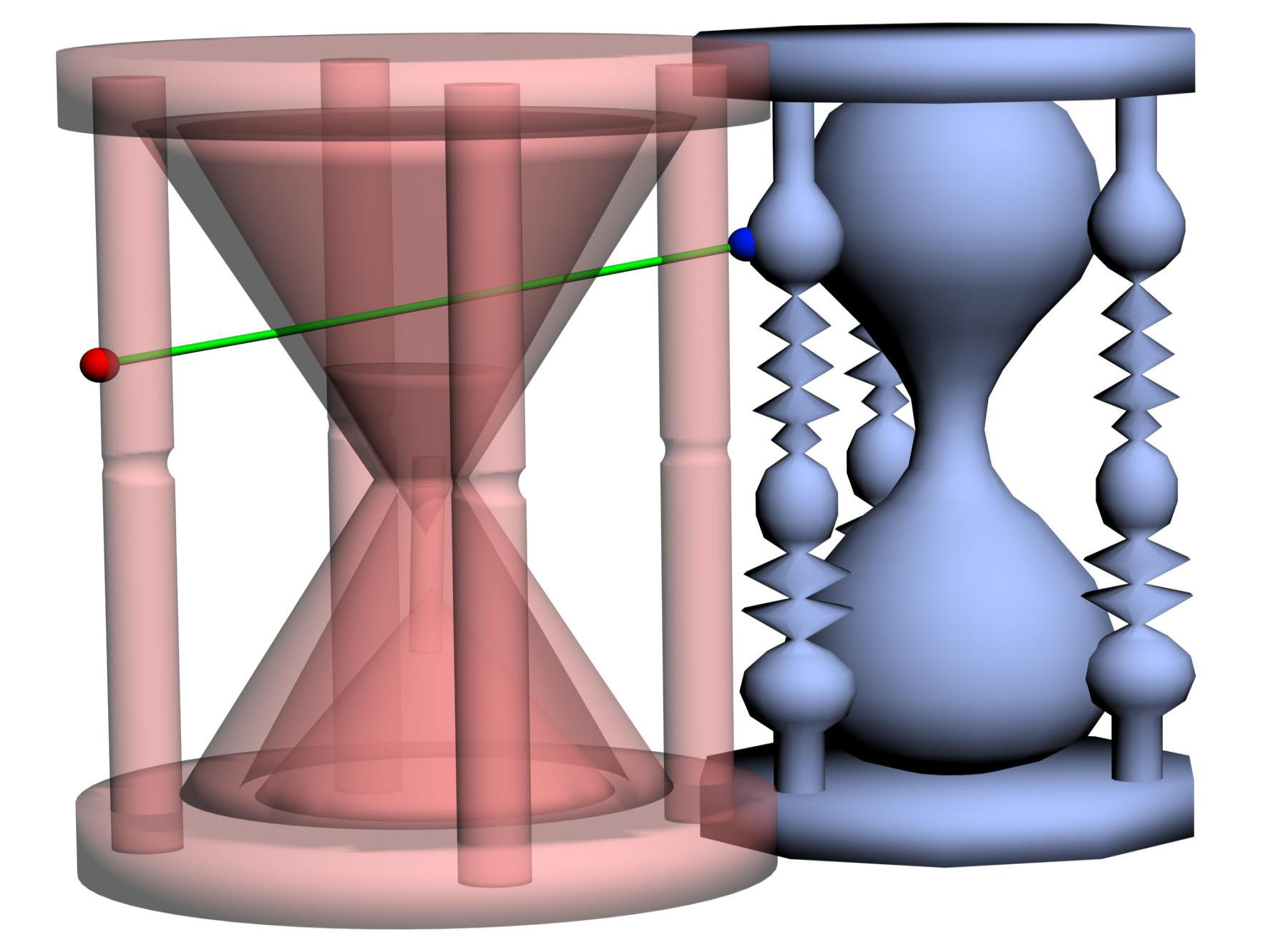Watch(3.8K) vs. Watch(38.1K)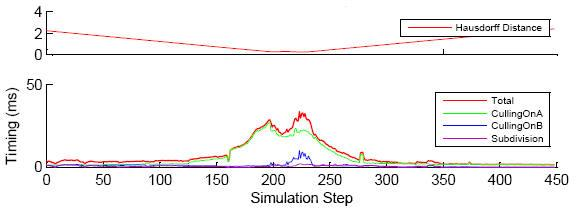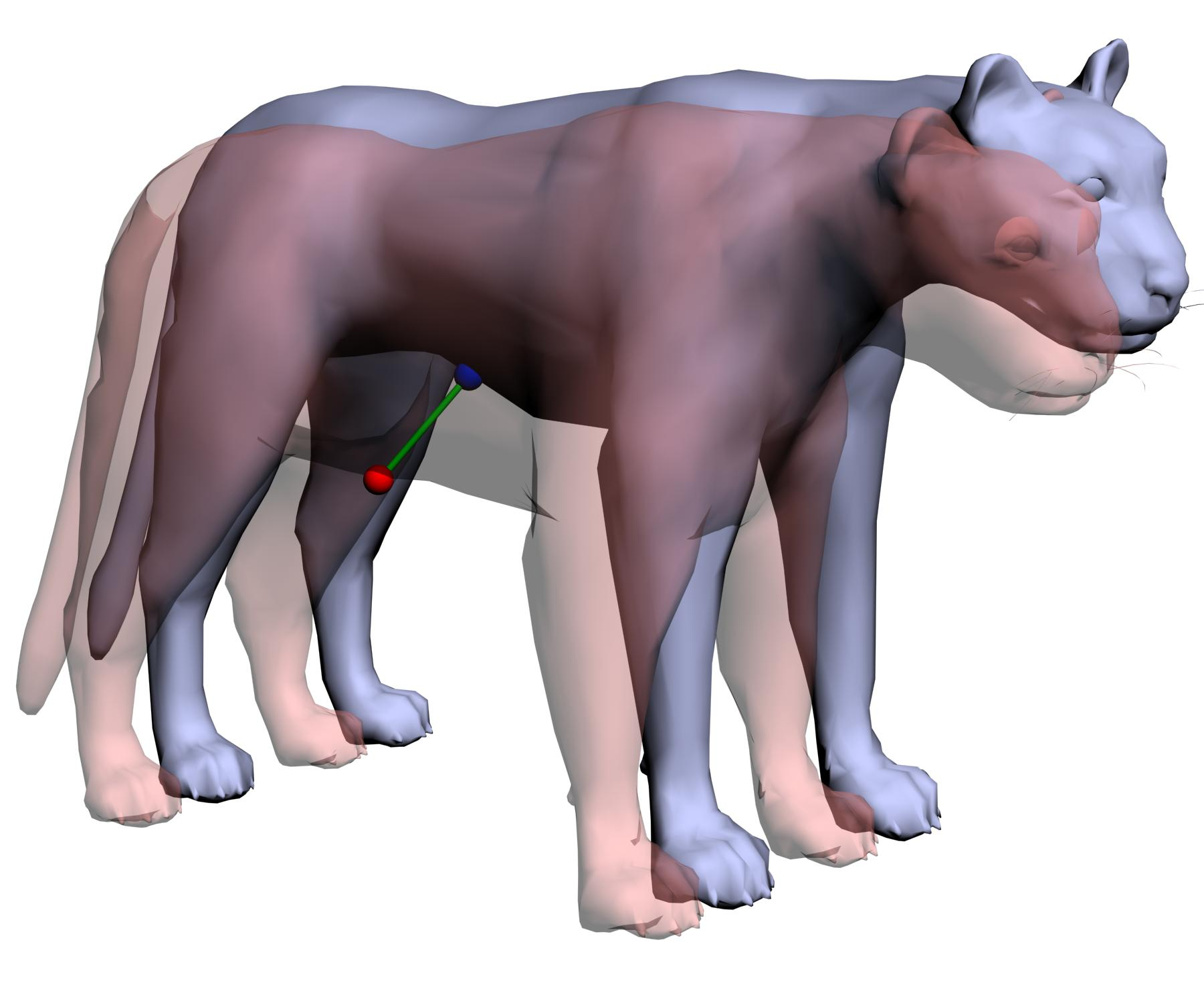Puma(8.2K) vs. Puma(7.1K)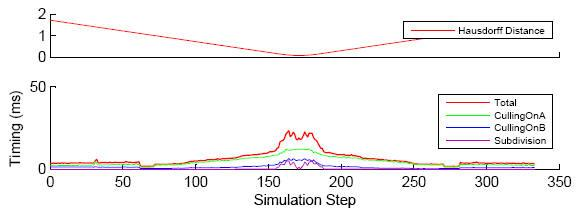(2) Penetration Depth Computation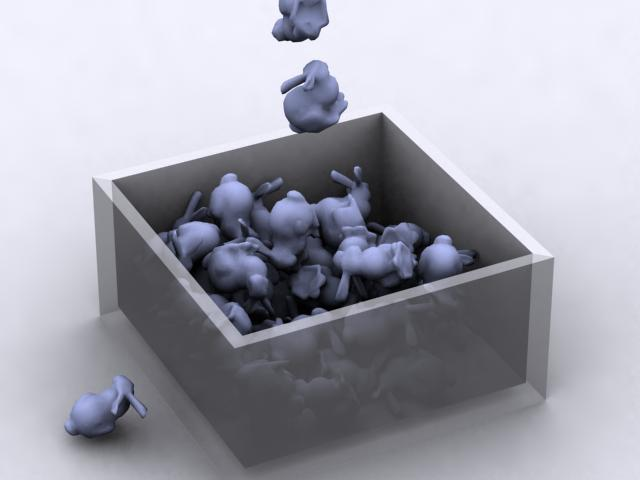Falling Bunnies scene we dropped 50 bunnies, each consisting of 1K triangles, on top of each other.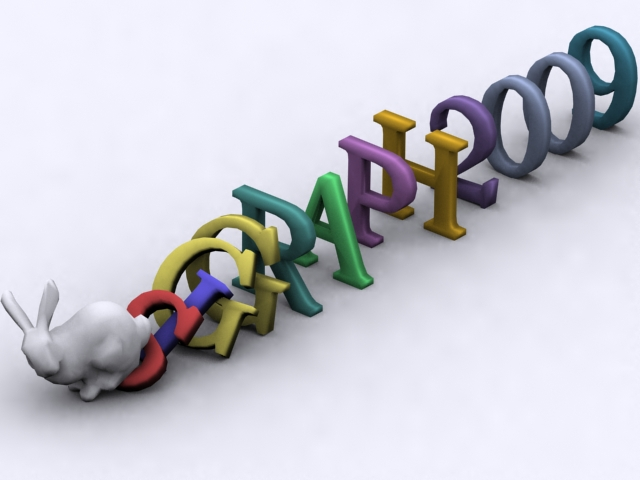Alphabet Domino scene we physically simulated the dominos consisting of 12 alphabet characters, each composed of around 200 triangles, and a bunny with 1K triangles.